# College Chemistry : Empirical and Molecular Formulas

## Example Questions

### Example Question #1 : Empirical And Molecular Formulas

What is the correct molecular formula for phosphoric acid?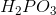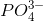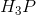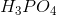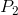Explanation:

Phosphoric acid combines a single phosphate ion with three hydrogen ions. The correct molecular formula is.is the molecular formula for hydrophosphoric acid,is the molecular formula for phosphorous acid, andis just a charged phosphate ion, which has biochemical significance with regards to DNA structure and energy molecules like ATP. Phosphorus is not a diatomic atom.

### Example Question #2 : Empirical And Molecular Formulas

A molecule with the empirical formula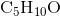has a molar mass of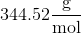. Find its molecular formula.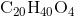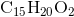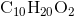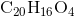Explanation:

Start by finding the molar mass of ofby adding up the molar masses of its constituent atoms.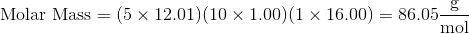Now, divide the molar mass of the molecule by the molar mass of its empirical formula.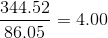In order to find the molecular formula, you will need to multiply the empirical formula by. Thus, the molecular formula is.

### Example Question #3 : Empirical And Molecular Formulas

What is the empirical formula for a compound that contains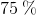carbon and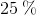hydrogen?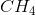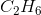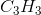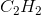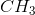Explanation:

We're given the percent composition of the elements carbon and hydrogen in a given compound, and we're asked to determine the empirical formula.

The first thing we have to do is determine the relative molar ratios of each element. In this case, because we are given the percent composition, we can assume that we're starting off with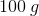of the starting material. That way, we can convert each percentage directly into grams.

Following that, we need to use the molar mass of each element in order to convert it from grams to moles.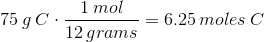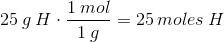In other words, what we have found is that in our compound, for every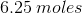of carbon, there will also be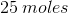of hydrogen.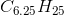But remember, the empirical formula for any given compound tells us the lowest whole number ratio of the compound's constituent elements. Therefore, we'll need to divide each value by the lowest number out of all the values available.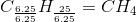What this tells us is that for every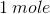of carbon in this compound, there will be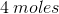of hydrogen as well. This is equivalent to the previous ratio, but now it has been reduced to the lowest possible whole numbers.